# All commands, alphabetic order; letter B

## \b

The \b command generates a bar-under accent. The translation of

\b B \b b \b D \b d \b h \b K \b k \b L \b l
\b N \b n \b R \b r \b T \b t \b Z \b z


is

&#7686; &#7687; &#7694; &#7695; &#7830; &#7732; &#7733; &#7738; &#7739;
&#7752; &#7753; &#7774; &#7775; &#7790; &#7791; &#7828; &#7829;


Preview:HTML characters Ḇ ḇ Ḏ ḏ ẖ Ḵ ḵ Ḻ ḻ Ṉ ṉ Ṟ ṟ Ṯ ṯ Ẕ ẕ

## \backepsilon (math symbol)

The \backepsilon command is valid only in math mode. It generates the reversed epsilon character <mo>&backepsilon;</mo> (Unicode 3F6, ϶).

## \backmatter

A command to be used after the main matter in a book. See description of the \mainmatter command.

## \backprime (math symbol)

The \backprime command is valid only in math mode. It generates the reversed prime character <mo>&backprime;</mo> (Unicode 2035, ‵).

## \backsim (math symbol)

The \backsim command is valid only in math mode. It generates the reversed tilde character <mo>&backsim;</mo> (Unicode 223D, ∽).

## \backsimeq (math symbol)

The \backsimeq command is valid only in math mode. It generates the reversed tilde with equals character <mo>&backsimeq;</mo> (Unicode 22CD, ⋍).

## \backslash (math symbol)

The \backslash command gives a backslash. It is valid only in math mode. Use \textbackslash for a backslash in text mode. Translation is <mo>&Backslash;</mo> (Unicode 2216, ∖). See also description of the \smallint command.

In TeX, the variable \badness contains the badness of the last box. In Tralics, you can say \count25=\badness if you want to put zero into count register 25, or you can say \the\badness if you want a zero digit.

## \bar

The \bar command puts a bar accent over a kernel. It works only in math mode; use the \= command outside math mode. See also the \acute command.

## \baro (math symbol)

The \baro command is valid in math mode only, it translates to <mo>&ovbar;</mo> (Unicode 233D, ⌽), it is a vertical bar in a circle.

## \barwedge (math symbol)

The \barwedge command is valid in math mode only, it translates to <mo>&#x22BC;</mo> (Unicode 22BC, ⊼).

## \barnodeconnect

The command \barnodeconnect[d]{F}{T} connects nodes F and T using a bar of depth d. See \abarnodeconnect command for syntax and usage.

## \baselineskip (rubber length)

You can say \baselineskip=10pt plus 2pt minus 3pt. This explains to TeX that it should put 10pt (maybe up to 2pt more, maybe up to 3pt less) of glue between the baselines of two consecutive lines in a paragraph (this may depend on \lineskiplimit). Unused by Tralics. (See scanglue for details of argument scanning).

## \baselinestretch

This commands expands to 1. (See TLC2, section 3.1.13).

## \batchmode

You can prefix the command \batchmode with \global. Nothing happens, since batch-mode is the only interaction mode.

## \bauthors, \beditors (Tralics command)

The two commands \bauthors and \beditors translate their argument, and put the result is a element of the same name. See \citation for details. These commands are produced when translating the bibliography, in this case the argument is a list of \bpers commands.

## \Bbbk

The \Bbbk command produces a blackboard k, it is the same as \mathbb{k}.

## \because (math symbol)

The \because command is valid in math mode only, it translates to <mo>&because;</mo> (Unicode U+2235, ∵).

## \begin

You can say something like \begin{something} some code \end{thesame}. The result of this is to translate the code inside a group. Moreover, some specific action is done at the beginning and the end of the environment. For instance \something is called first, and \endthesame is called at the end.

The documentation says that \begin{em} some code \end{em} is the same as {\em some code}. Practically, this means that \end{thesame} produces nothing (in particular no error), if \endthesame is not defined.

The argument to \begin and \end is converted to a command name via \csname, hence macros are expanded. If you say \def\xx#1{}\begin{\xx}, you get the following LateX error: ! Missing \endcsname inserted. <to be read again> \relax. This is because the command \xx reads the \endcsname marker, and checking whether the environment is defined inserts a \relax. For the same example Tralics says End of data reached while expecting \endcsname, and in the case of \begin{abc\par cde}, the error reads Invalid token \par found while scanning \begin. No error is signaled in the case \begin{abc\endcsname cde}. In both these cases, the environment is named abc and the superfluous tokens cde are ignored.

If you say: \begin{\em} ... \end{\em}, LaTeX complains with ! Missing \endcsname inserted. This is because \em is a robust command (one for which expansion can be delayed); it expands to \protect which cannot be expanded (the meaning of \protect depends on the context).

If you say \def\FOO{foo} \begin{\FOO}\end{foo} you get the following error: ! LaTeX Error: \begin{foo} on input line 22 ended by \end{foo}. This is because the names are different \FOO versus foo, and the error message is printed via \errmessage that expands everything. No error is signaled by Tralics in this case. Note the funny error for \begin{a}\end{a}, because \a tries to fetch an accent: ! LaTeX Error: Command \\end unavailable in encoding T1. Note that \begin{}\end{} gives infinite recursion in LaTeX, this is a special case in Tralics. The commands \begin{group} \end{group} give two errors: first Undefined environment group then Extra \endgroup found in unclosed environment group.

Historical note. Before 2.13.3, only characters were allowed in an environment name (nothing is expanded). Since version 2.10, spaces are allowed in an environment name. Since version 2.14, environment names can have less than two letters.

Environments should nest properly. What this means is unclear to me. You may say \newenvironment{foo} {\begin{bar}} {\end{bar}} followed by \begin{foo} ... \end{foo}. When the \end command is seen, the current environment is bar' and the argument is foo': these names are not the same. However \endfoo will call \end{bar} and close the other environment. As a consequence, only a posteriori tests can be made. This makes error recovery unclear.

## \@begintheorem

Private Tralics command.

## \beginL, \beginR

These commands are ε-TeX extensions that indicate the start of a left-to-right or right-to-left region; they are ignored by Tralics.

## \begingroup

The \begingroup command defines a semi-simple group, that must be terminated by \endgroup. In some cases a construct like \begingroup\it x\endgroup is exactly the same as {\it x}, or as \bgroup\it x\egroup.

## \belowdisplayshortskip (rubber length)

You can say \belowdisplayshortskip=10pt plus 2pt minus 3pt. The \belowdisplayshortskip register contains a skip value that TeX puts after a short display. The value is unused by Tralics. (See scanglue for details of argument scanning). (See \predisplaysize for further details).

## \belowdisplayskip (rubber length)

You can say \belowdisplayskip=10pt plus 2pt minus 3pt. The \belowdisplayskip register contains a skip value that TeX puts after a display. The value is unused by Tralics. (See scanglue for details of argument scanning). (See \predisplaysize for further details).

## Beqnarray, Beqnarray* (environments)

The fancybox package provides a variant of the eqnarray environment that can be used inside a box; the documentation provides this example:


\fbox{%
\begin{Beqnarray*}
x &=&y\\
y& >& x\\
\int_4^5 f(x)dx &=&\sum_{i\in F} x_i
\end{Beqnarray*}
}


Here is the translation. The math formula consists in a table with three rows, omitted here.

<fbox rend='boxed'>
<formula textype='Beqnarray*' type='display'>
<math mode='display' xmlns='http://www.w3.org/1998/Math/MathML'>
<mtable displaystyle='true'>
<mtr>...</mtr><mtr>...</mtr><mtr>...</mtr>
</mtable>
[/itex]
</formula>
</fbox>


## \beta (math character)

The \beta command is valid only in math mode. It generates a Greek letter: <mi>&beta;</mi> (Unicode U+3B2, β) that renders like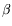. See description of the \alpha command.

## \beth (math character)

The \beth command is valid only in math mode. It generates a Hebrew letter: <mi>&beth;</mi> (Unicode U+2136, ℶ).

## \between (math symbol)

The \between command is valid only in math mode. It generates a symbol: <mi>&between;</mi> (Unicode U+226C, ≬).

## \bezier

This is like \qbezier but it has a first required argument instead of optional. See the \qbezier command.

## \bf

The \bf command is equivalent to \normalfont\bfseries. In other words, it selects a font of roman family, bold series and upright shape. For an example of fonts, see \rm.

## \bfseries

The \bfseries command changes the series of the current font to a bold face series. For an example of fonts, see \rm.

## \bgroup

The \bgroup command is an open brace of catcode 1. For an example see catcode1.

## \bibitem

This command takes two arguments, the first being optional. Translation of \bibitem [toto] {titi} is <bibitem id='bid17' bib-key='toto'/>. The element will have an id and a bib-key attribute that comes from the optional argument. If you say \cite{titi}, this will create a reference to this bibitem element. (See \XMLsolvecite for more details). Note that the equivalent of \noindent is executed; this implies that a new paragraph is started. [Translation changed in version 2.12.1]

## \bibitem@empty (Tralics command)

You can say \bibitem@empty{foo}, this will signal an error because the command has been withdrawn.

## \bibliography

The \bibliography command takes as argument a list of file-names from which bibtex takes the bibliographical entries. In Tralics, this command has as side-effect to load the bibliography, i.e. the bbl file. In the current version, this file is created by Tralics and not by an external program. Example: here is the bib file. After saying \nocite{*}, Tralics generated this bbl file.

## \bibliographystyle

When you say \bibliographystyle{alpha}, this tells bibtex to use the alpha.bst file. In the current version, Tralics does not call bibtex, and the bibliography is handled, independently of the argument of this command. In a future version, something will be done.

## \big, \Big, \bigg, \Bigg

The \big declaration is valid only in math mode. It is ignored unless what follows is a character that can become bigger in the vertical dimension. In some cases, \big is replaced by a \left or \right command. Hence all four commands \big \Big \bigg \Bigg produce the same result. If you say \big(, the result is an opening delimiter, that can be converted into \left(; if you say \bigl), the result is also an opening delimiter, because the command says so. If you say \bigm), you get a middle delimiter, that can produce a \middle( (the \middle command is not yet implemented). For an example, see \mathord.

## \bigl, \Bigl, \biggl, \Biggl

See \big above. This is a left (opening) delimiter.

## \bigr, \Bigr, \biggr, \Biggr

See \big above. This is a right (closing) delimiter.

## \bigm, \Bigm, \biggm, \Biggm

See \big above. This is a middle (neither opening nor closing) delimiter.

## \bigbox (math symbol)

The \bigbox command is valid only in math mode. It is an alias for \square. Translation is <mo>&square;</mo> (Unicode U+25A1, □)

## \bigbreak

In Tralics, the \bigbreak command is the same as \par. In LaTeX, it makes also sure that the space between the two paragraphs is at least \bigskipamount, by calling \bigskip if necessary; not implemented in Tralics.

## \bigcap (math symbol)

The \bigcap command is valid only in math mode. Its translation is a variable-sized symbol (used for set intersection): <mo>&bigcap;</mo> (Unicode U+22C2, ⋂) that renders like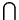. For an example see the \sum command.

## \bigcirc (math symbol)

The \bigcirc command is valid only in math mode. It generates a binary operator (big circle): <mo>&bigcirc;</mo> (Unicode U+25EF, ◯) that renders like. See description of the \pm command.

## \bigcircle

The \bigcircle command is defined by the curves package. See \arc.

## \bigcup (math symbol)

The \bigcup command is valid only in math mode. Its translation is a variable-sized symbol (used for set union): <mo>&bigcup;</mo> (Unicode U+22C3, ⋃) that renders like. For an example see the \sum command.

## \bigcurlyvee (math symbol)

The \bigcurlywedge command is valid only in math mode. It is an alias to \curlyvee.

## \bigcurlywedge (math symbol)

The \bigcurlywedge command is valid only in math mode. It is an alias to \curlywedge.

## \bignplus (math symbol)

The \bignplus command is valid only in math mode. It is an alias to \nplus. Note these two commands are described but not implemented.

## \biginterleave (math symbol)

The \biginterleave command is valid only in math mode. It is an alias to \interleave.

## \bigodot (math symbol)

The \bigodot command is valid only in math mode. Its translation is a variable-sized symbol (dot in a circle): <mo>&bigodot;</mo> (Unicode U+2A00, ⨀) that renders like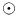. For an example see the \sum command.

## \bigoplus (math symbol)

The \bigoplus command is valid only in math mode. Its translation is a variable-sized symbol (plus in a circle): <mo>&bigoplus;</mo> (Unicode U+2A01, ⨁) that renders like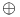. For an example see the \sum command.

## \bigotimes (math symbol)

The \bigotimes command is valid only in math mode. Its translation is a variable-sized symbol (times in a circle): <mo>&bigotimes;</mo> (Unicode U+2A02, ⨂) that renders like. For an example see the \sum command.

## \bigparallel (math symbol)

The \bigparallel command is valid only in math mode. It is an alias to \parallel.

## \bigskip

The \bigskip command is like the \smallskip command. (see also the \vskip command).

## \bigskipamount (rubber length)

This is defined by Tralics in the same way as in LaTeX, but is unused, see \smallskip

## \bigsqcap (math symbol)

The \bigsqcap command is valid only in math mode. Its translation is a variable-sized symbol (square cap): <mo>&sqcap;</mo> (Unicode U+2293, ⊓). For an example see the \sum command.

## \bigsqcup (math symbol)

The \bigsqcup command is valid only in math mode. Its translation is a variable-sized symbol (square cup): <mo>&bigsqcup;</mo> (Unicode U+2A06, ⨆). For an example see the \sum command.

## \bigstar (math symbol)

The \bigstar command is valid only in math mode. Its translation is <mo>&bigstar;</mo> (Unicode U+2605, ★).

## \bigtriangledown (math symbol)

The \bigtriangledown command is valid only in math mode. It generates a binary operator. Looks like a reversed \Delta: <mo>&bigtriangledown;</mo> (Unicode U+25BD, ▽) that renders like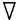. See description of the \pm command.

## \bigtriangleup (math symbol)

The \bigtriangleup command is valid only in math mode. It generates a binary operator. Looks like a \Delta: <mo>&bigtriangleup;</mo> (Unicode U+25B3, △) that renders like. See description of the \pm command.

## \biguplus (math symbol)

The \biguplus command is valid only in math mode. Its translation is a variable-sized symbol. It is like a plus in a cup: <mo>&biguplus;</mo> (Unicode U+228E, ⊎) that renders like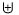. For an example see the \sum command.

## \bigvee (math symbol)

The \bigvee command is valid only in math mode. Its translation is a variable-sized symbol. It looks like a big V: <mo>&bigvee;</mo> (Unicode U+22C1, ⋁) that renders like. For an example see the \sum command.

## \bigwedge (math symbol)

The \bigwedge command is valid only in math mode. Its translation is a variable-sized symbol. It looks like a big reversed V: <mo>&bigwedge;</mo> (Unicode U+22C0, ⋀) that renders like. For an example see the \sum command.

## \binampersand (math symbol)

The \binampersand command is valid only in math mode. Its translation is <mo>&amp;</mo>.

## \bindnasrepma (math symbol)

The \bindnasrepma command is valid only in math mode. Its translation is <mo>&#x214B;</mo>

## \binom

The \binom command is valid in math mode only. It could be defined as \def\binom{\genfrac()\z@{}}. The translation is a <mfence> element, containing a <mfrac> element. The two arguments of the \binom command are typeset one above the other, with parentheses around. There are two variants \dbinom \tbinom that control the size of the arguments. Note that \left(\right) produces bigger parentheses than \genfrac. Example.


\def\n{\mathchoice{a}{b}{c}{d}}
$$\binom{\frac{\n+m}{2}} \n \quad \tbinom{\frac{\n+m}{2}} \n \quad \dbinom{\frac{\n+m}{2}} \n\qquad {}^{\binom{\frac{\n+m}{2}} \n \quad \tbinom{\frac{\n+m}{2}} \n \quad \dbinom{\frac{\n+m}{2}} \n}$$


This is the translation. Comments were not generated by Tralics

<formula type='display'>
<math xmlns='http://www.w3.org/1998/Math/MathML'>
<mrow><mfenced open='(' close=')'>
<!--\binom{\frac{\n+m}{2}} \n -->
<mfrac linethickness='0.0pt'>
<mfrac>
<mrow><mi>c</mi><mo>+</mo><mi>m</mi></mrow> <mn>2</mn></mfrac>
<mi>b</mi>
</mfrac>
</mfenced>
<mspace width='1.em'/>
<!--\tbinom{\frac{\n+m}{2}} \n -->
<mfenced open='(' close=')'>
<mstyle scriptlevel='0' displaystyle='false'>
<mfrac linethickness='0.0pt'>
<mfrac><mrow><mi>d</mi><mo>+</mo><mi>m</mi></mrow> <mn>2</mn></mfrac>
<mi>c</mi>
</mfrac>
</mstyle>
</mfenced>
<mspace width='1.em'/>
<!--\dbinom{\frac{\n+m}{2}} \n -->
<mfenced open='(' close=')'>
<mstyle scriptlevel='0' displaystyle='true'>
<mfrac linethickness='0.0pt'>
<mfrac><mrow><mi>c</mi><mo>+</mo><mi>m</mi></mrow> <mn>2</mn></mfrac>
<mi>b</mi>
</mfrac>
</mstyle>
</mfenced>
<mspace width='2.em'/>
<msup>
<mrow></mrow>
<mrow>
<!-- {}^\binom{\frac{\n+m}{2}} \n -->
<mfenced open='(' close=')'>
<mfrac linethickness='0.0pt'>
<mfrac><mrow><mi>d</mi><mo>+</mo><mi>m</mi></mrow> <mn>2</mn></mfrac>
<mi>d</mi>
</mfrac>
</mfenced>
<mspace width='1.em'/>
<!-- {}^\tbinom{\frac{\n+m}{2}} \n -->
<mfenced open='(' close=')'>
<mstyle scriptlevel='0' displaystyle='false'>
<mfrac linethickness='0.0pt'>
<mfrac><mrow><mi>d</mi><mo>+</mo><mi>m</mi></mrow> <mn>2</mn></mfrac>
<mi>c</mi>
</mfrac>
</mstyle>
</mfenced>
<mspace width='1.em'/>
<!--{}^\dbinom{\frac{\n+m}{2}} \n -->
<mfenced open='(' close=')'>
<mstyle scriptlevel='0' displaystyle='true'>
<mfrac linethickness='0.0pt'>
<mfrac><mrow><mi>c</mi><mo>+</mo><mi>m</mi></mrow> <mn>2</mn></mfrac>
<mi>b</mi>
</mfrac>
</mstyle>
</mfenced>
</mrow>
</msup>
</mrow>
[/itex]
</formula>


Preview:(See also here).

## \binoppenalty (integer parameter)

If you say \binoppenalty=26, then TeX will use 26 as penalty for line break in a math formula after a binary operator (like +). This parameter is ignored by Tralics (note that, in the current implementation, $a+b$ and $a\mathrel+b$ are identically translated; it is hence impossible to decide whether the plus is binary or not). (See scanint for details of argument scanning).

## \blacklozenge (math symbol)

The \blacklozenge command is valid only in math mode. Its translation is <mo>&blacklozenge</mo> (Unicode U+29EB, ⧫).

## \blacksquare (math symbol)

The \blacksquare command is valid only in math mode. Its translation is <mo>&blacksquare;</mo> (Unicode U+25AA, ▪).

## \blacktriangle (math symbol)

The \blacktriangle command is valid only in math mode. Its translation is <mo>&blacktriangle;</mo> (Unicode U+25B4, ▴)

## \blacktriangledown (math symbol)

The \blacktriangledown command is valid only in math mode. Its translation is <mo>&blacktriangledown;</mo> (Unicode U+25BE, ▾).

## \blacktriangleleft (math symbol)

The \blacktriangleleft command is valid only in math mode. Its translation is <mo>&blacktriangleleft;</mo> (Unicode +U25C2, ◂).

## \blacktriangleright (math symbol)

The \blacktriangleright command is valid only in math mode. Its translation is <mo>&blacktriangleright;</mo> (Unicode U+25B8, ▸).

## \bmod

This command is one the 4 modulo commands. Here is an example (TLC2, 8-4-7, first half).

\begin{align*}
u& \equiv v+1 \mod{n^2} \\
u& \equiv v+1 \bmod{n^2} \\
u&   =    v+1 \pmod{n^2} \\
u&   =    v+1 \pod{n^2} \\
\end{align*}


This is the translation

<formula id-text='mid1' id='uid1' type='display'>
<math mode='display' xmlns='http://www.w3.org/1998/Math/MathML'>
<mtable displaystyle='true'>
<mtr>
<mtd columnalign='right'><mi>u</mi></mtd>
<mtd columnalign='left'><mrow><mo>&equiv;</mo><mi>v</mi><mo>+</mo><mn>1</mn>
<mspace width='3.33333pt'/><mo form='prefix'>mod</mo><mspace width='0.277778em'/>
<msup><mi>n</mi> <mn>2</mn> </msup></mrow></mtd>
</mtr>
<mtr>
<mtd columnalign='right'><mi>u</mi></mtd>
<mtd columnalign='left'><mrow><mo>&equiv;</mo><mi>v</mi><mo>+</mo><mn>1</mn>
<mspace width='0.277778em'/><mo form='prefix'>mod</mo><mspace width='0.277778em'/>
<msup><mi>n</mi> <mn>2</mn> </msup></mrow></mtd>
</mtr>
<mtr>
<mtd columnalign='right'><mi>u</mi></mtd>
<mtd columnalign='left'><mrow><mo>=</mo><mi>v</mi><mo>+</mo><mn>1</mn>
<mspace width='10.0pt'/><mo>(</mo><mo form='prefix'>mod</mo><mspace width='0.277778em'/></mrow>
<msup><mi>n</mi> <mn>2</mn> </msup><mo>)</mo></mrow></mtd>
</mtr>
<mtr>
<mtd columnalign='right'><mi>u</mi></mtd>
<mtd columnalign='left'><mrow><mo>=</mo><mi>v</mi><mo>+</mo><mn>1</mn>
<mspace width='10.0pt'/><mo>(</mo><msup><mi>n</mi> <mn>2</mn> </msup><mo>)</mo></mrow>
</mtd>
</mtr>
</mtable>
[/itex]
</formula>


Preview. (See also here).

## body (Tralics environment)

The body environment is defined as \newenvironment{body}{}{} in versions up to 2.3 for compatibility reasons. It is now a user-defined environment for the raweb only. It is undefined otherwise.

## \boolean

See \newboolean for how to create a boolean and \ifthenelse for how to use it with the \boolean{some-name} command.

## \bot (math symbol)

The \bot command is valid only in math mode. It generates a miscellaneous symbol. It is like \perp: <mo>&bottom;</mo> (Unicode U+22A5, ⊥) that renders like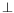. See description of the \ldots command.

## \botfigrule

The command \botfigrule behaves like \relax, in LaTeX it can be redefined to produce a rule between a float and text that precedes.

## \botmark

In \botmark is the mark text most recently encountered on the page that was just boxed. Since Tralics does not box pages, the expansion of \botmark is always empty.

## \botmarks

This is an extension introduced by ε-TeX; an integer is read, expansion is empty. See \splitbotmark.

## \bottom (math symbol)

The \bottom command is an alias for \bot.

## \bottomfraction

Maximum fraction of a page that can be occupied by floats at the bottom. Unused by Tralics. See \intextsep.

## bottomnumber (counter)

Maximum number of floats at the bottom of a page. Unused by Tralics. See \intextsep.

## \bowtie (math symbol)

The \bowtie command is valid only in math mode. It generates a relation symbol: <mo>&bowtie;</mo> (Unicode U+22C8,⋈) that renders like See description of the \le command.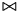## \Box (math symbol)

The \Box command is valid only in math mode. It generates a square box <mo>&25A1;</mo>. There is the image of x\Box.

## \box

The \box command reads an integer N (a small number between 0 and 255, see scanint for details), and returns the content of the box number N. After that, the box is emptied (the \copy command behaves in the same fashion, but leaves the box unchanged). Example

\setbox12=\xbox{foo}{ok}
\count3=12
\copy12 , and \copy 12 , and \box \count3 , and \box 12!


Translation

<foo>ok</foo>, and <foo>ok</foo>, and <foo>ok</foo>, and !


## \boxast (math symbol)

The \boxast command is valid only in math mode. It generates a box with asterisk <mo>&#x29C6;</mo> (Unicode U+29C6, ⧆).

## \boxbox (math symbol)

The \boxbox command is valid only in math mode. It generates a box with a box <mo>&29C8;</mo> (Unicode U+29C8, ⧈).

## \boxbslash (math symbol)

The \boxbslash command is valid only in math mode. It generates a box with a backslash <mo>&bsolb;</mo> (Unicode U+29C5, ⧅).

## \boxcircle (math symbol)

The \boxcircle command is valid only in math mode. It generates a box with <mo>&#x29C7;</mo> (Unicode U+29C7, ⧇).

## \boxdot (math symbol)

The \boxdot command is valid only in math mode. It generates a box with a dot <mo>&dotsquare;</mo> (Unicode U+22A1, ⊡).

## \boxed

The \boxed command is valid only in math mode. It takes an argument, and puts a frame around it (like fbox in text mode); as the example below shows, the argument is put in a cell of a table, and the table has a frame attribute. (See also here).

<mtable frame='solid'><mtr><mtd><mi>x</mi></mtd></mtr></mtable>


## \boxempty (math symbol)

The \boxempty command is valid only in math mode. It generates an empty box. It is an alias for \square. Translation is <mo>&square;</mo> (Unicode U+25A1, □).

## \boxmaxdepth (rigid length)

You can say \boxmaxdepth=1.5in, as a result TeX will make sure that each vbox on a page has a depth not exceeding 1.5in (by shifting it up if necessary). Ignored by Tralics. (See scandimen for details of argument scanning).

## \boxminus (math symbol)

The \boxminus command is valid only in math mode. It generates a box with a minus sign <mo>&boxminus;</mo> (Unicode U+229F, ⊟).

## \boxplus (math symbol)

The \boxplus command is valid only in math mode. It generates a box with a plus sign <mo>&boxplus;</mo> (Unicode U+229E, ⊞).

## boxslash\ (math symbol)

The \boxslash command is valid only in math mode. It generates a box with slash <mo>&solb;</mo> (Unicode U+29C4, ⧄).

## \boxtimes (math symbol)

The \boxtimes command is valid only in math mode. It generates a box with product sign <mo>&boxtimes;</mo> (Unicode U+22A0, ⊠).

## \bpers (Tralics command)

This command is used for an author or editor in a bibliography. It takes one optional and four required arguments (first name, initials, particle, last name, junior name). For an example, see \citation. Here the arguments of the command are \bpers[David]{D.}{}{Carlisle}{}, from the database value 'David Carlisle'. The translation is a <bpers> element, with five attributes (in the case of particle and junior, no attribute is created if the field is empty, so that the effective number of attributes is between three and five). An example is <bpers prenom='D.' nom='Carlisle' prenomcomplet='David'/>.

## \bprime (math symbol)

The \bprime command is valid only in math mode. It generates <mo>&bprime;</mo> (Unicode U+2035).

## \brace, \brack (math construction)

Instead of {a\brace b} or {a\brack b} you should use \genfrac\{\}{0pt}{}{a}{b} and \genfrac[]{0pt}{}{a}{b}.

## \bracevert (math symbol)

The \bracevert command is valid only in math mode. It is an alias for \vert.

## \break

The \break command is ignored. It is \penalty-10000 in LaTeX.

## \breve

The \breve command puts a breve accent over a kernel. It works only in math mode. See also the \acute command.

## \brokenpenalty (internal integer)

When you say \brokenpenalty=98, then TeX will use 98 as penalty for a page break at a discretionary break (case where a word is broken, the two parts being on two different pages). Unused by Tralics. (See scanint for details of argument scanning).

## \Bumpeq (math character)

The \Bumpeq command is valid only in math mode. It generates <mo>&Bumpeq;</mo> (Unicode U+224E, ≎).

## \bullet (math character)

The \bullet command is valid only in math mode. It generates a binary operator: <mo>&bullet;</mo> (Unicde U+2022, •) See description of the \pm command.

## \bye

This is defined in PlainTeX as : \par \vfill \supereject \end. In Tralics, we make it equivalent to \endinput.back to home page © INRIA 2003-2005, 2006, 2008 Last modified $Date: 2015/11/27 17:06:15$